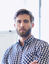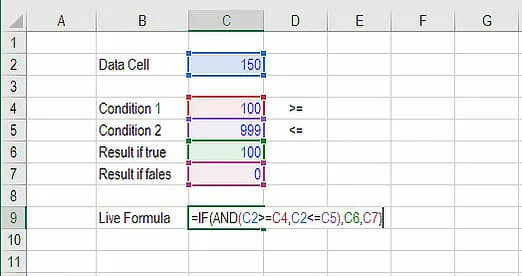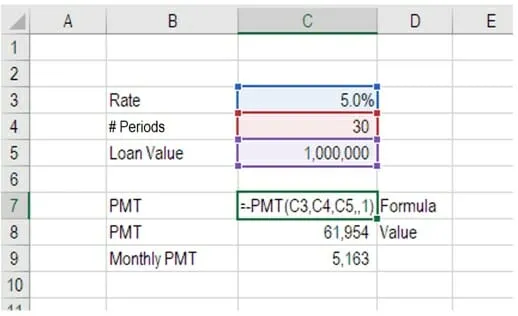Search
•James Richard

# The Most Powerful Excel Functions You Aren’t Using

Updated: Apr 12If you’re reading this, you likely work in corporate finance. While it isn’t possible to dissect everything about your personality and capabilities from that one facet of your professional life, it is certain to say that you possess some competency with Microsoft Excel. Sure, your line of work may involve other tools that are more high tech and “up-to-date”, but you likely still use Excel some, and at least had to rely on for one extended period of time in your professional experience. Given the years you have spent working with this continuously relevant application, you probably feel pretty good about yourself in regards to knowing the most effective functions in Excel. However, with this post, I want to flesh out 7 of the most effective formulas in the application that you will hopefully start taking advantage of, if you aren’t using them already.

## 7 Excel Functions You Should Be Using

### 1. INDEX MATCH

Formula: =INDEX(C3:E9,MATCH(B13,C3:C9,0),MATCH(B14,C3:E3,0))

This is a relatively advanced alternative to the VLOOKUP or HLOOKUP formulas (which have several drawbacks and limitations). INDEX MATCH is a powerful combination of Excel formulas that will take your financial analysis and financial modeling to the next level.

INDEX returns the value of a cell in a table based on the column and row number.

MATCH returns the position of a cell in a row or column.

Here is an example of the INDEX and MATCH formulas combined together. In this example, a person’s height based on their name is looked up and returned. Since name and height are both variables in the formula, both of them can be changed.### 2. OFFSET combined with SUM or AVERAGE

Formula: =SUM(B4:OFFSET(B4,0,E2-1))

The OFFSET function on its own is not particularly advanced, but when combined with other functions like SUM or AVERAGE, it makes for a relatively sophisticated formula. Suppose you want to create a dynamic function that can sum a variable number of cells. With the regular SUM formula, you are constricted to a static calculation, but by adding OFFSET you can have the cell reference move around.

How it works: To make this formula work, the ending reference cell of the SUM function is substituted with the OFFSET function. This makes the formula dynamic and the cell referenced as E2 is where you can tell Excel how many consecutive cells you want to add up.

The following is an example of this slightly more sophisticated formula in action.### 3. IF combined with AND / OR

Formula: =IF(AND(C2>=C4,C2<=C5),C6,C7)

Anyone who’s spent a great deal of time doing various types of financial models knows that nested IF formulas can be a nightmare. Combining IF with the AND or the OR function can be a great way to keep formulas easier to audit and easier for other users to understand. In the example below, you will see how to use individual functions in combination to create a more advanced formula.### 4. XNPV and XIRR

Formula: =XNPV(discount rate, cash flows, dates)

If you’re an analyst working in investment banking, equity research, financial planning & analysis (FP&A), or any other area of corporate finance that requires discounting cash flows, then these formulas are a lifesaver!

Simply put, XNPV and XIRR enable you to apply specific dates to each individual cash flow that’s being discounted. The problem with Excel’s basic NPV and IRR formulas is that they assume the time periods between cash flow are equal. Routinely, as an analyst, you’ll encounter situations where cash flows are not timed evenly, and this formula is how you fix that.### 5. CHOOSE

Formula: =CHOOSE(choice, option1, option2, option3)

The CHOOSE function is great for scenario analysis in financial modeling. It enables you to pick between a specific number of options, and return the “choice” that you’ve selected. For example, imagine you have three different assumptions for revenue growth next year: 5%, 12%, and 18%. Using the CHOOSE formula you can return 12% if you tell Excel you want choice #2.### 6. SUMIF and COUNTIF

Formula: =COUNTIF(D5:D12,”>=21″)

These two advanced formulas are great uses of conditional functions. SUMIF adds all cells that meet certain criteria, and COUNTIF counts all cells that meet certain criteria. For example, imagine you want to count all cells that are greater than or equal to 21 (the legal U.S. drinking age ) to find out how many bottles of champagne you need for a client event. You can use COUNTIF as an advanced solution, as shown in the example below.### 7. PMT and IPMT

Formula: =PMT(interest rate, # of periods, present value)

If you work in commercial banking, real estate, FP&A or any financial analyst position that deals with debt schedules, you’ll want to understand these two detailed formulas.

The PMT formula gives you the value of equal payments over the life of a loan. You can use it in conjunction with IPMT (which tells you the interest payments for the same type of loan), then separate principal and interest payments.

Here is an example of how to use the PMT function to get the monthly mortgage payment for a \$1 million mortgage at 5% for 30 years.### Moving Forward with Excel Skills in The New Year

In the realm of corporate finance, it is of course common to continue using Excel alongside other software, because of how confident and familiar so many people feel with the use of this application. However, don’t be so sure of your competency if you’re not proficient in all of these functions; you can seriously seize a critical advantage by amplifying your abilities with this perennial application.